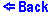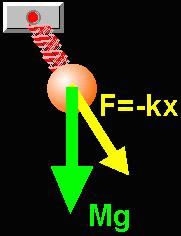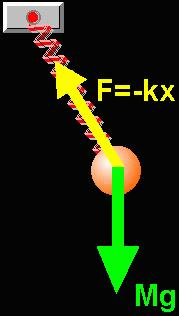The Spring Pendulum
One can drag the pendulum to it's starting position.
Once in motion, the pendulum can be 'caught' by clicking and holding the mass.This applet shows the two dimensional spring pendulum: a mass suspended on a spring. The pendulum moves under the influence of gravity and the elastic force of the spring. The apparently simple motion of this system is complex enough that no equations exist that would describe it's path. The motion of the system is calculated using Newton's Second Law: a = Fnet / M. The net force is the vector sum of the gravitational force Mg and the elastic force of the spring F = - k x.